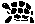« 2019-12-29 Tinkering 2019-05-26 »

# Tinkering: 2019-12-17: Radius Gauge for Calipers

Another small tool to measure a radius with normal calipers. The tool aligns the caliper so that it is easy to measure the radius. What the calipers really measure is the distance between the circumference of a corner and the imaginary edge of the corner if the corner was angled at 90°. To compute the radius of the corner from this, the reading of the calipers needs to be multiplied by sqrt(2)+1 (or alternatively, divided by sqrt(2)-1).

Why is the math like that? Well, that's homework!

Also homework: how is multiplying by X identical to dividing by X-2?

Anyway, here are some photos.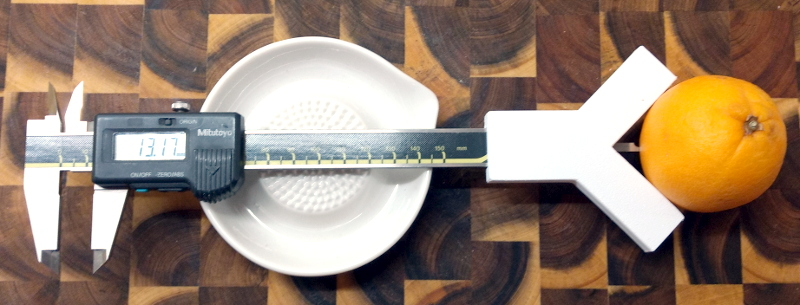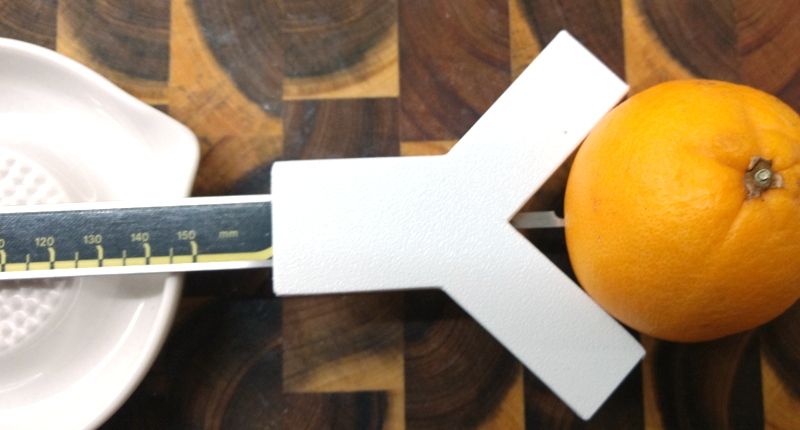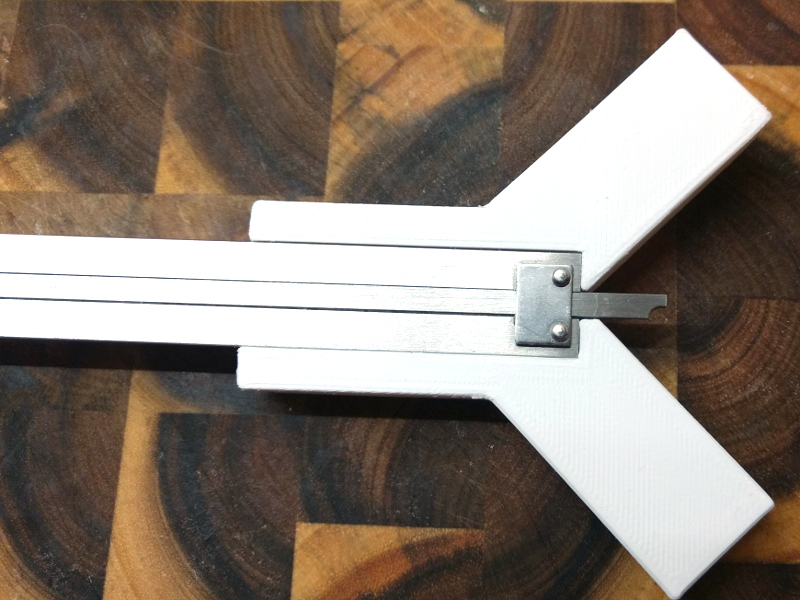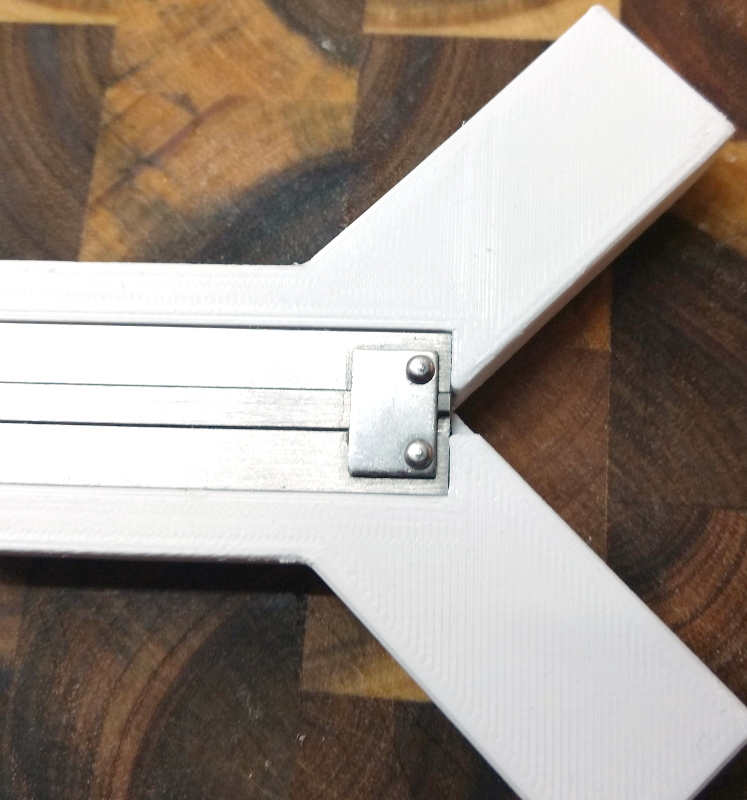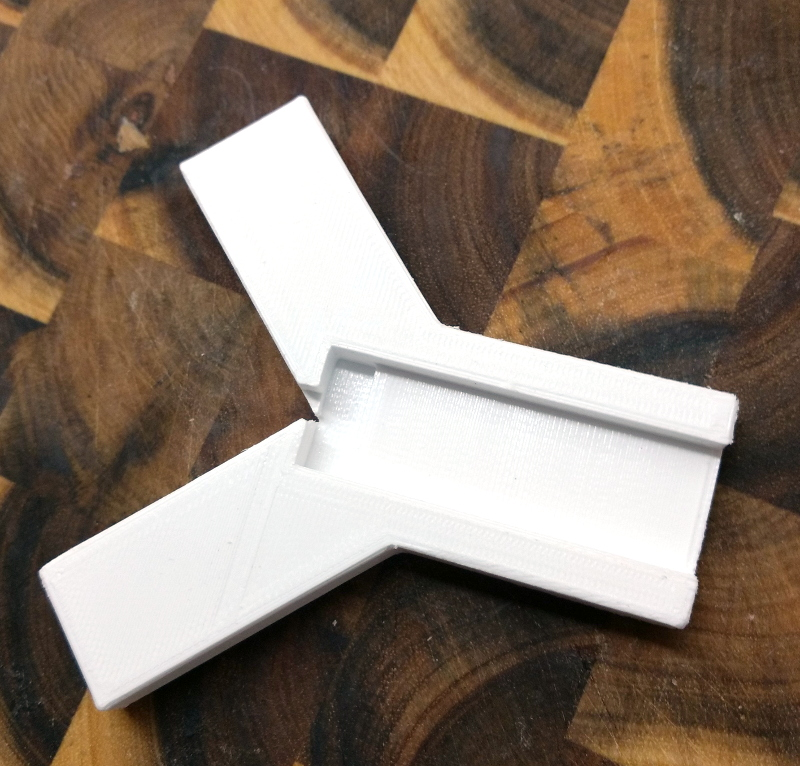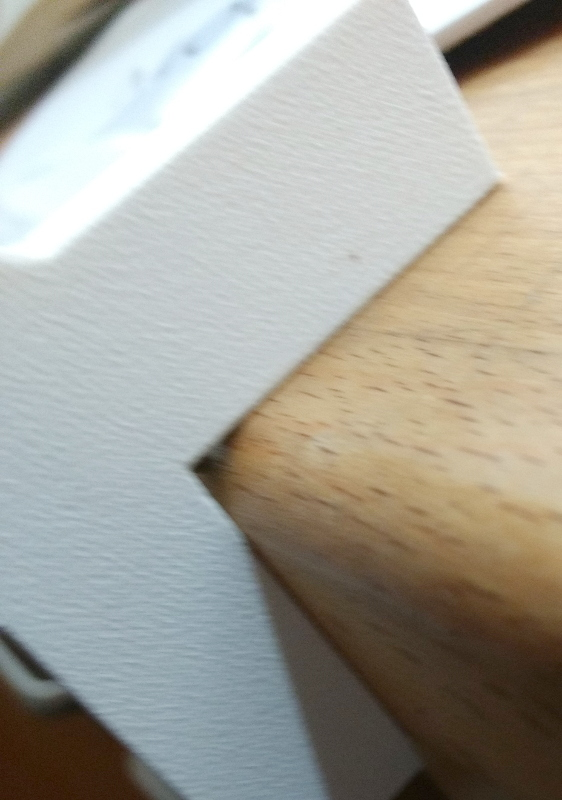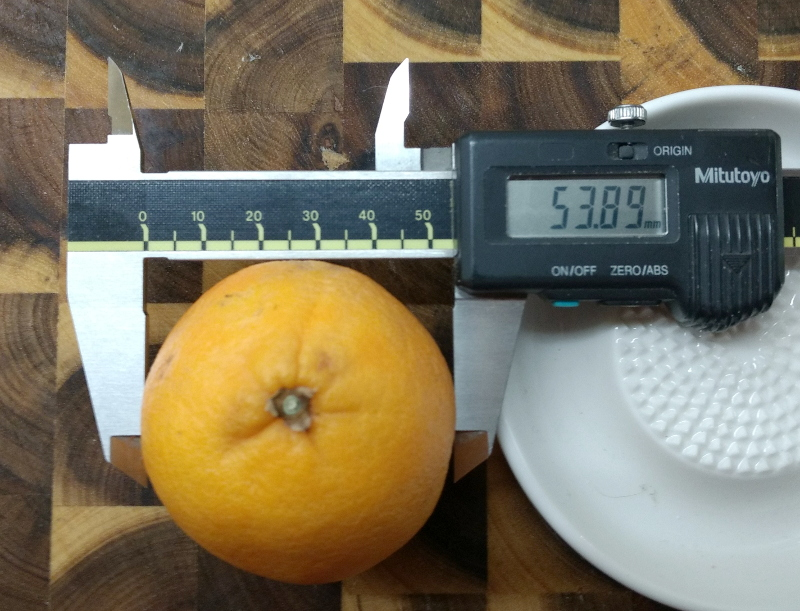OK, so why does it read '13.17' and the diameter is actually '53.89' when actually, it should be 13.17 * (sqrt(2)+1) * 2 = 63.59? Well, the orange I used was not very round. Sorry.

To get good precision, after printing the insides of the thighs of the Y should be sanded or filed down a bit until the caliper hits a pointed square angle. The plastic usually comes out a bit squashed, so this is never immediately accurate.

I measured wooden corners at 2.65mm which, when multipled by sqrt(2)+1 is 6.4mm, and the actual radius is 1/4 inch = 6.35mm. This is pretty good. 8mm corners registered at 3.35mm, which comes out of the calculation as 8.09mm. This is also quite OK, I think.

```radgaug.zip